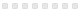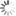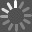# Symmetric Automorphisms of Free Products

Unknown - 1996
Rate this:This memoir examines the automorphism group of a group \$G\$ with a fixed free product decomposition \$G_1*\cdots *G_n\$. An automorphism is called symmetric if it carries each factor \$G_i\$ to a conjugate of a (possibly different) factor \$G_j\$. The symmetric automorphisms form a group \$\Sigma Aut(G)\$ which contains the inner automorphism group \$Inn(G)\$. The quotient \$\Sigma Aut(G)/Inn(G)\$ is the symmetric outer automorphism group \$\Sigma Out(G)\$, a subgroup of \$Out(G)\$. It coincides with \$Out(G)\$ if the \$G_i\$ are indecomposable and none of them is infinite cyclic. To study \$\Sigma Out(G)\$, the authors construct an \$(n-2)\$-dimensional simplicial complex \$K(G)\$ which admits a simplicial action of \$Out(G)\$. The stabilizer of one of its components is \$\Sigma Out(G)\$, and the quotient is a finite complex. The authors prove that each component of \$K(G)\$ is contractible and describe the vertex stabilizers as elementary constructs involving the groups \$G_i\$ and \$Aut(G_i)\$. From this information, two new structural descriptions of \$\Sigma Aut (G)\$ are obtained. One identifies a normal subgroup in \$\Sigma Aut(G)\$ of cohomological dimension \$(n-1)\$ and describes its quotient group, and the other presents \$\Sigma Aut (G)\$ as an amalgam of some vertex stabilizers. Other applications concern torsion and homological finiteness properties of \$\Sigma Out (G)\$ and give information about finite groups of symmetric automorphisms. The complex \$K(G)\$ is shown to be equivariantly homotopy equivalent to a space of \$G\$-actions on \$\mathbb R\$-trees, although a simplicial topology rather than the Gromov topology must be used on the space of actions.
Publisher: Providence, R.I. : American Mathematical Society, 1996
ISBN: 9780821804599
0821804596
Branch Call Number: QA3 .A57 no. 582
Characteristics: p. ; cm## Opinion

### From the critics### Community Activity

#### Comment

There are no comments for this title yet.

#### Age

There are no ages for this title yet.

#### Summary

There are no summaries for this title yet.

#### Notices

There are no notices for this title yet.

#### Quotes

There are no quotes for this title yet.## Explore FurtherLoading...Loading...